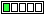All about flooble | fun stuff | Get a free chatterbox | Free JavaScript | Avatarsperplexus dot infoA Quicky III (Posted on 2014-04-09)If each of the a,b,c,d is a decimal digit - for how many ordered quadruplets (a,b,c,d) is the sum S= a+b+6c+d divisible by 3 ?Comments: ( Back to comment list | You must be logged in to post comments.)How many are divisible by 1?| Comment 3 of 9 |Well, let me solve the easier problem of just counting how many ordered quadruplets there are.

If all the digits are the same, then there are 10 quadruplets.

If there are only two different digits, then C(10,2)*3 = 135
The 3 multiplier is because the digits (1,2) can be either (1,1,1,2) or (1,1,2,2) or (1,2,2,2).

If there are three different digits, then C(10,3)*3 = 360
The 3 multiplier is because the digits (1,2,3) can be either (1,1,2,3) or (1,2,2,3) or (1,2,3,3).

If there are four different digits, then C(10,4) = 210.

Total quadruplets = 10 + 135 + 360 + 210 = 715

 Posted by Steve Herman on 2014-04-09 12:14:36Please log in:

 Search: Search body:
Forums (0)